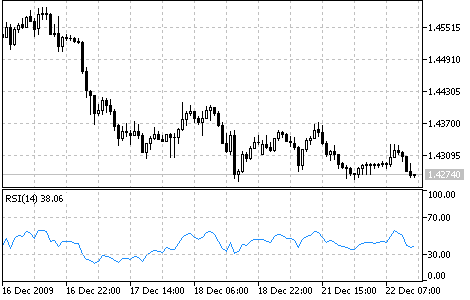Interesting script?
So post a link to it -
let others appraise it

You liked the script? Try it in the MetaTrader 5 terminalRelative Strength Index (RSI) - indicator for MetaTrader 5

Views:
15768
Rating:
Published:
2010.01.26 11:22
Updated:
2016.11.22 07:32

The Relative Strength Index technical indicator (RSI) is a price-following oscillator that ranges between 0 and 100. When Wilder introduced the Relative Strength Index, he recommended using a 14-day RSI.. Since then, the 9-day and 25-day Relative Strength Index indicators have also gained popularity.

A popular method of analyzing the RSI is to look for a divergence in which the security is making a new high, but the RSI is failing to surpass its previous high. This divergence is an indication of an impending reversal. When the Relative Strength Index then turns down and falls below its most recent trough, it is said to have completed a "failure swing". The failure swing is considered a confirmation of the impending reversal.

Ways to use Relative Strength Index for chart analysis:

• Tops and bottoms The Relative Strength Index usually tops above 70 and bottoms below 30. It usually forms these tops and bottoms before the underlying price chart;
• Chart Formations The RSI often forms chart patterns such as head and shoulders or triangles that may or may not be visible on the price chart;
• Failure swing (Support or Resistance penetrations or breakouts) This is where the Relative Strength Index surpasses a previous high (peak) or falls below a recent low (trough);
• Support and Resistance levels The Relative Strength Index shows, sometimes more clearly than price themselves, levels of support and resistance.
• Divergences As discussed above, divergences occur when the price makes a new high (or low) that is not confirmed by a new high (or low) in the Relative Strength Index. Prices usually correct and move in the direction of the RSI.Calculation:

The Relative Strength index is calculated by formula:

RSI = 100 - (100 / (1 + U / D))

where:

• U - is the average number of positive price changes;
• D - is the average number of negative price changes.

Translated from Russian by MetaQuotes Software Corp.
Original code: https://www.mql5.com/ru/code/47Price Rate of Change (ROC)

The Price Rate of Change (ROC) reflects this ondulatory movement like an oscillator, measuring the difference in prices in a certain period. ROC grows if prices grow and drops along with them. The more the price change is, the more ROC changes.Price and Volume Trend (PVT)

The Price and Volume Trend Indicator(PVT), like On Balance Volume (OBV), represents the cumulative sum of trade volumes calculated considering close price changes.Relative Vigor Index (RVI)

The main point of the Relative Vigor Index Indicator (RVI) is that on the bull market the closing price is, as a rule, higher, than the opening price.Standard Deviation (StdDev)

The Standard Deviation (StdDev) measures the market volatility. This indicator charactrizes the scale of price changes relating to the Moving Average.